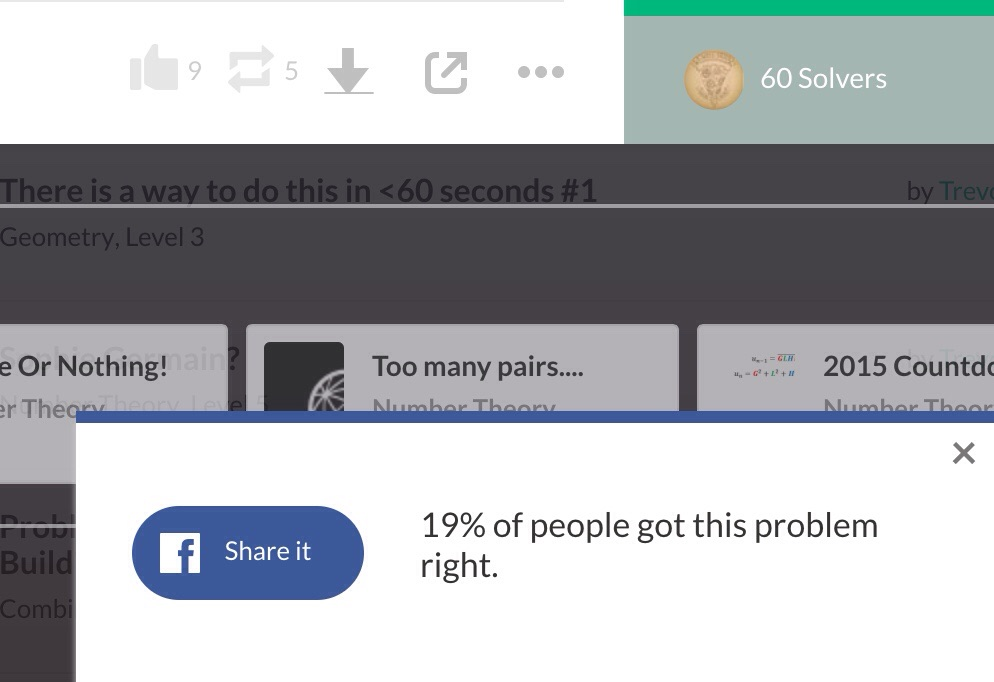# %solversBy now, you must have seen that little rectangle pop up in the bottom corner of the brilliant problem pages saying "X% of people solved this problem."

Now, assume that the % solvers is determined by $\lfloor \frac{\text{\# of solvers}}{\text{total views}}\cdot 100\rfloor$

Where $\lfloor x \rfloor$ denotes the floor function of the greatest integer less than or equal to x)

What is the minimum number of views that a problem can have if the % solvers displayed is 19%.

This is part of the set Trevor's Ten

×